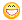About Store Forum Documentation ContactProblem with code not working Author Message
DandruffMemberPost: #1 Problem with code not working I have a randomly generated side-view of a landscape (think of Terraria). It is 2048x768 and it would then be used to create a wall of 1x1x1 cubes. Box creator Code: ```if(land.lock()) // in order to edit the texture we must first lock it       {          FREPD(   x, land.x()) // iterate through all x's          {             // db++;  this ran 2048 times, as expected             Int mhy=mhcalcy(x);  // calculate max height of y on cur x                          // creates the boxes from an earlier declared array of Actor box             box[x].create(Box(1, 1, 1, Vec(x, mhy, 0)), 0);                          land.color(x,mhy,Color(GREEN));                      }       }``` Problem is, when i launch it and pan around, i get from the first x cube to the supposed last x cube (last i can see) faster than i expected. I then checked it to make sure if there were indeed 2048 cubes (drawn). Checker Code: ```if(Kb.b(KB_H))    {       Int interval;       interval+=Time.d(); //thanks Driklyn! :)              static Int current;              if(interval>=0.4f)       {          terrain.db++;          Cam.setPosDir(Vec(terrain.box[current++].pos().x,terrain.box[current++].pos().y, terrain.box[current++].pos().z-15));          interval=0;       }    }``` After hitting cube ~650, the Cam jumped from looking at the cubes to looking at 0,0 (so there's actually around that much cubes drawn, not 2048). Holding it just for fun, it hit cube 827 and crashed, probably from accessing an element that's out of range. Any help is appreciated. Thanks! image of landscapeimage of first boxes seenthen it just ends abruptly08-11-2011 06:40 AM
DriklynMember

 Post: #2 RE: Problem with code not working In checker, shouldn't interval be of type Flt, not Int? Also, you should always manually initialize local variables before using them. Not doing so can result in unexpected behavior. Your code may work one day and have a bug the next (because it won't always necessarily be initialized to 0, could be some other random number). Code: `Flt interval = 0;` And don't you want to call current++ just once? Since you're calling it three times, your loop will end three times faster (2048 / 3 = ~682, which is close to the ~650 you mention in your post). Code: ```Cam.setPosDir(Vec(terrain.box[current].pos().x,terrain.box[current].pos().y,terrain.box[current].pos().z-15)); current++;``` ---------- Thanks for the mention in your post!That was surprising to see!08-11-2011 08:05 AM
DandruffMemberPost: #3 RE: Problem with code not working Ahh right, thanks. I guess there are 2048 cubes!08-11-2011 08:50 PM
 « Next Oldest | Next Newest »